Anzeige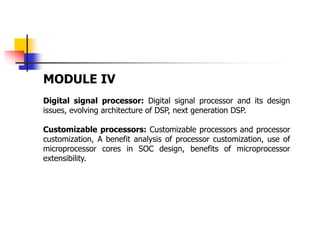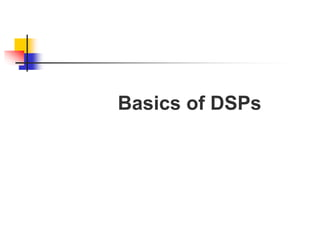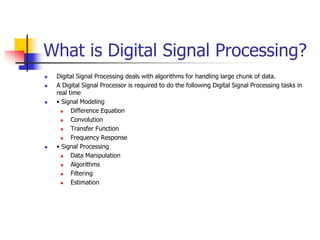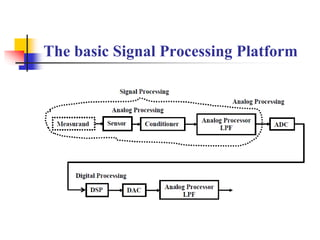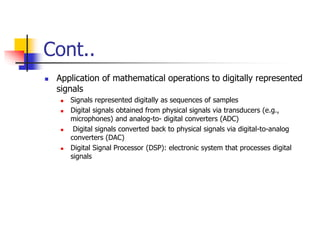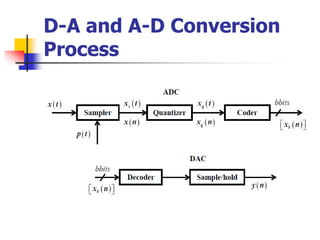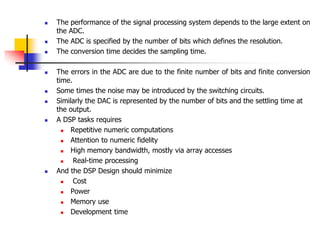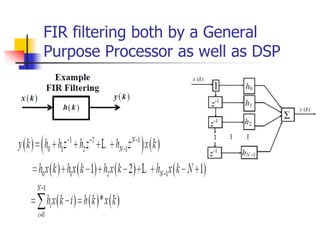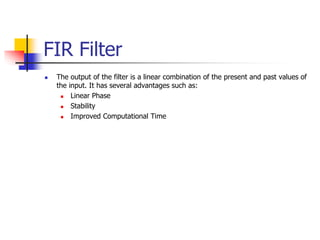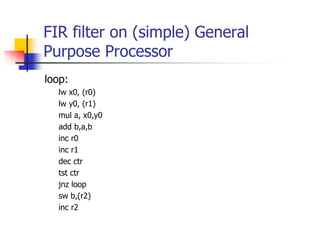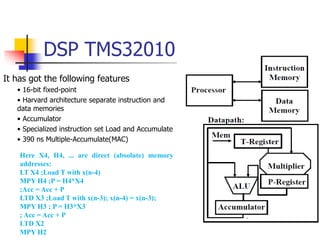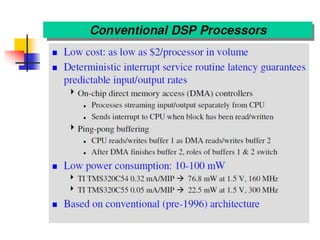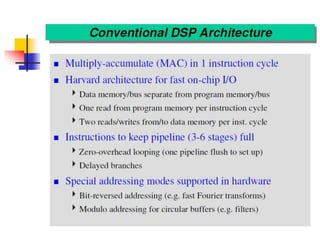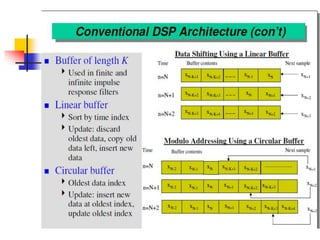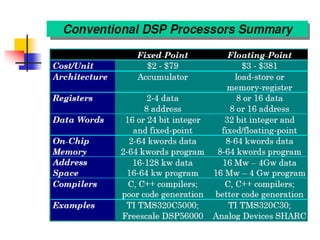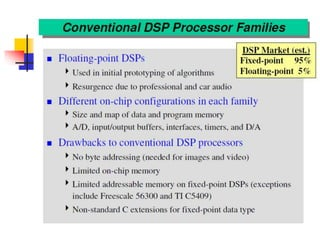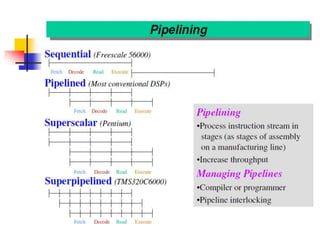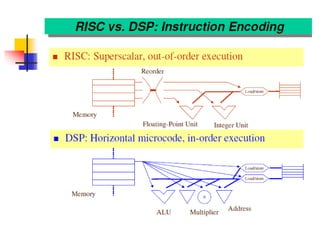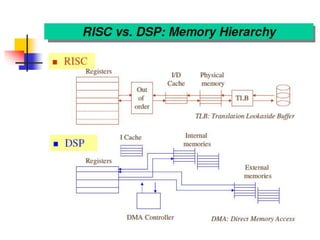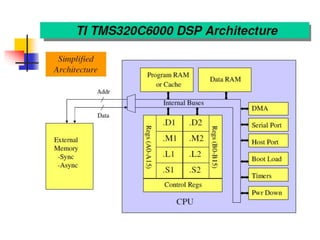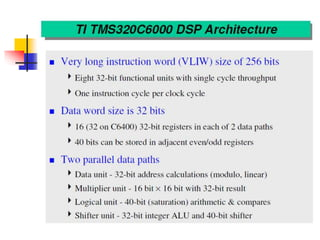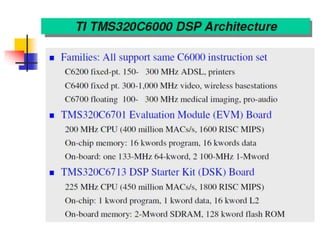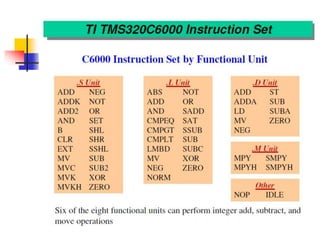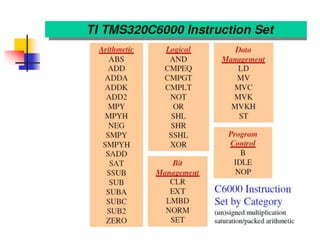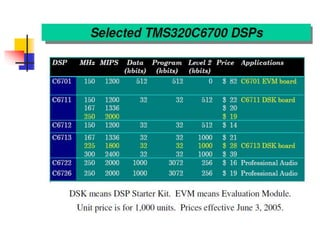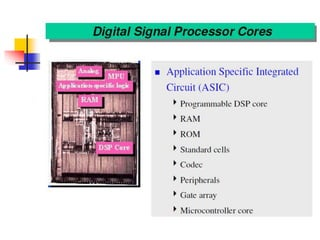1 von 26
Anzeige

### Lect1a_ basics of DSP.pptx

1. MODULE IV Digital signal processor: Digital signal processor and its design issues, evolving architecture of DSP, next generation DSP. Customizable processors: Customizable processors and processor customization, A benefit analysis of processor customization, use of microprocessor cores in SOC design, benefits of microprocessor extensibility.
2. Basics of DSPs
3. What is Digital Signal Processing?  Digital Signal Processing deals with algorithms for handling large chunk of data.  A Digital Signal Processor is required to do the following Digital Signal Processing tasks in real time  • Signal Modeling  Difference Equation  Convolution  Transfer Function  Frequency Response  • Signal Processing  Data Manipulation  Algorithms  Filtering  Estimation
4. The basic Signal Processing Platform
5. Cont..  Application of mathematical operations to digitally represented signals  Signals represented digitally as sequences of samples  Digital signals obtained from physical signals via transducers (e.g., microphones) and analog-to- digital converters (ADC)  Digital signals converted back to physical signals via digital-to-analog converters (DAC)  Digital Signal Processor (DSP): electronic system that processes digital signals
6. D-A and A-D Conversion Process
7.  The performance of the signal processing system depends to the large extent on the ADC.  The ADC is specified by the number of bits which defines the resolution.  The conversion time decides the sampling time.  The errors in the ADC are due to the finite number of bits and finite conversion time.  Some times the noise may be introduced by the switching circuits.  Similarly the DAC is represented by the number of bits and the settling time at the output.  A DSP tasks requires  Repetitive numeric computations  Attention to numeric fidelity  High memory bandwidth, mostly via array accesses  Real-time processing  And the DSP Design should minimize  Cost  Power  Memory use  Development time
8. FIR filtering both by a General Purpose Processor as well as DSP
9. FIR Filter  The output of the filter is a linear combination of the present and past values of the input. It has several advantages such as:  Linear Phase  Stability  Improved Computational Time
10. FIR filter on (simple) General Purpose Processor loop: lw x0, (r0) lw y0, (r1) mul a, x0,y0 add b,a,b inc r0 inc r1 dec ctr tst ctr jnz loop sw b,(r2) inc r2
11. DSP TMS32010 It has got the following features • 16-bit fixed-point • Harvard architecture separate instruction and data memories • Accumulator • Specialized instruction set Load and Accumulate • 390 ns Multiple-Accumulate(MAC) Here X4, H4, ... are direct (absolute) memory addresses: LT X4 ;Load T with x(n-4) MPY H4 ;P = H4*X4 ;Acc = Acc + P LTD X3 ;Load T with x(n-3); x(n-4) = x(n-3); MPY H3 ; P = H3*X3 ; Acc = Acc + P LTD X2 MPY H2
Anzeige